Click in-app to access the full platform documentation for your version of DataRobot.

# Use the ROC Curve tools¶

The ROC Curve tools provide visualizations and metrics to help you determine whether the classification performance of a particular model meets your specifications. It is important to understand that the ROC Curve and other charts on that tab are based on a sample of calculated thresholds. That is, DataRobot calculates thresholds for all data and then, because sampling provides faster performance (returns results in the UI more quickly), it uses a maximum of 120 thresholds—a quantile-based representative selection—for the visualizations. Manual calculations are slightly more precise, therefore, the initial auto-generated calculations and the manually generated will not match exactly.

Benefits of ROC curve visualizations

Models produce probabilities, values anywhere from 0 to 1, with millions if not 10s or 100s of millions of unique possibilities. The action you take based on model results, however, is binary—you either send an offer or you don’t, for example. To "convert" all those probabilities to a binary action, you select a threshold (“any probability above X% and I send the offer”). The threshold creates two values—false positives and true positives, which can be plotted on a graph. The number of false and true positives vary as you change your threshold. A very low threshold results in a lot of false positives, whereas a high threshold provides none.

Conceptually, the best outcome (and what a good model will give you) is low false positives and high true positives. If you look at an ROC curve, points in the upper left are what you want, as they represent low false positive, high true positive. A model that does no better than a coin flip will show a 45-degree line.

## Access the ROC Curve tools¶

1. To access the ROC Curve tab, navigate to the Leaderboard, select the model you want to evaluate, then click Evaluate > ROC Curve. The ROC Curve tab contains the set of interactive graphical displays described below.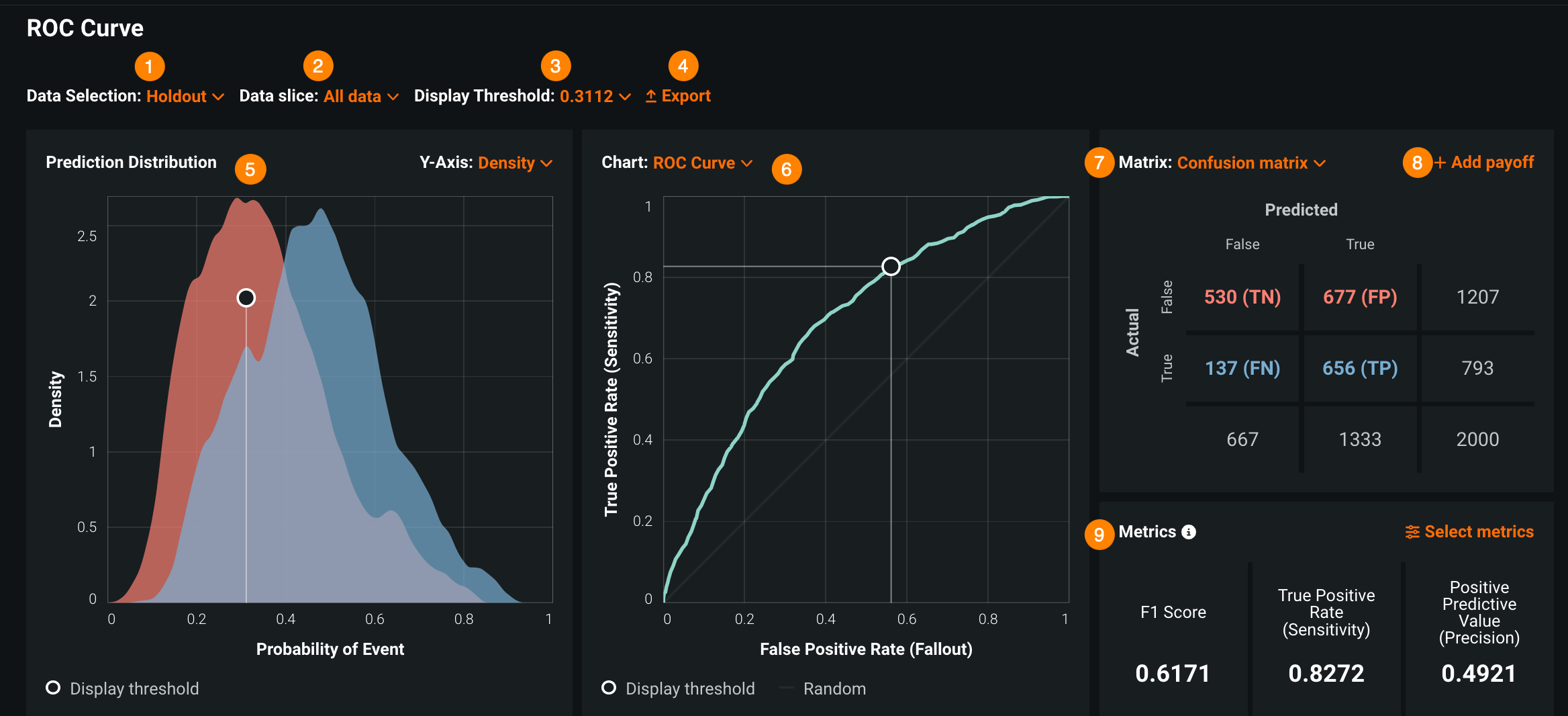Tip

This visualization supports sliced insights. Slices allow you to define a user-configured subpopulation of a model's data based on feature values, which helps to better understand how the model performs on different segments of data. See the full documentation for more information.

Element DescriptionData Selection Select the data source for your visualization. Data sources can be partitions—Holdout, Cross Validation, and Validation—as well as external test data. Once you select a data source, the ROC curve visualizations update to reflect the new data.Data slice Binary classification only. Selects the filter that defines the subpopulation to display within the insight.Display Threshold Select a display threshold that separates predictions classified as "false" from predictions classified as "true."Export Export to a CSV, PNG, or ZIP file:
• Download a PNG of a ROC Curve, Profit Curve, Prediction Distribution graph, Cumulative Gain chart, or a Cumulative Lift chart.
• Download a ZIP file containing all of the CSV and PNG files.Prediction Distribution Use the Prediction Distribution graph to evaluate how well your classification model discriminates between the positive and negative classes. The graph separates predictions classified as "true" from predictions classified as "false" based on the prediction threshold you set.Chart selector Select a type of chart to display. Choose from ROC Curve (default), Average Profit, Precision Recall, Cumulative Lift (Positive/Negative), and Cumulative Gain (Positive/Negative). You can also create your own custom chart.Matrix selector Select a type of matrix to display. By default, a confusion matrix displays. You can choose to display the confusion matrix data by instance counts or percentages. You can instead create a payoff matrix so that you can generate and view a profit curve.+ Add payoff Enter payoff values to generate a profit curve so that you can estimate the business impact of the model. Clicking Add payoff displays a Payoff Matrix in the Matrix pane if not already displayed. Adjust the Payoff values in the matrix and set the Chart pane to Average Profit to view the impact.Metrics View summary statistics that describe model performance at the selected threshold. Use the Select metrics menu to choose up to six metrics to display at one time.
2. To use these components, select a data source and a display threshold between predictions classified as "true" or "false"—each component works together to provide an interactive snapshot of the model's classification behavior based on that threshold.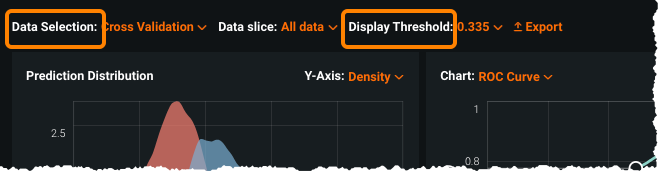Note

Several Wikipedia pages and the Internet in general provide thorough descriptions explaining many of the elements provided by the ROC Curve tab. Some are summarized in the sections that follow.

## Classification use cases¶

The following sections use one of two binary classification use cases to illustrate the concepts described. In both cases, each row in the dataset represents a single patient, and the features (columns) contain descriptive variables about the patient's medical condition.

The ROC curve is a graphical means of illustrating classification performance for a model as the relevant performance statistics at all points on the probability scale change. To understand the reported statistics, you must understand the four possible outcomes of a classification problem; these outcomes are the basis of the confusion matrix.

### Classification use case 1¶

Use case 1 asks "Does a patient have diabetes?" This hypothetical dataset has both categorical and numeric values and describes whether a patient has diabetes. The target variable, `has_diabetes`, is a categorical value that describes whether the patient has the disease (`has_diabetes=1`) or does not have the disease (`has_diabetes=0`). Numeric and other categorical variables describe factors like blood pressure, payer code, number of procedures, days in hospital, and more. For use case 1:

Outcome Description
True positive (TP) A positive instance that the model correctly classifies as positive. For example, a diabetic patient correctly identified as diabetic.
False positive (FP) A negative instance that the model incorrectly classifies as positive. For example, a healthy patient incorrectly identified as diabetic.
True negative (TN) A negative instance that the model correctly classifies as negative. For example, a healthy patient correctly identified as healthy.
False negative (FN) A positive instance that the model incorrectly classifies as negative. For example, a diabetic patient incorrectly identified as healthy.

The following points provide some statistical reasoning behind using the outcomes:

• Correct predictions: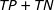• Incorrect predictions: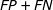• Total scored cases: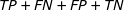• Error rate: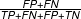• Overall accuracy (probability a prediction is correct):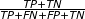### Classification use case 2¶

Use Case 2 is a model that tries to determine whether a diabetic patient will be readmitted to hospital (the target feature). This hypothetical dataset has both categorical and numeric values and describes whether a patient will be readmitted to the hospital within 30 days (target `variable=readmitted`). This categorical value describes whether the patient is readmitted inside of 30 days (`readmitted=1`) or is not readmitted within that time frame (`readmitted=0`); other categorical values include things like admission id and payer code. Numeric variables describe things like blood pressure, number of procedures, days in hospital, and more.

Note

DataRobot displays the ROC Curve tab only for models created for a binary classification target (a target with two unique values).

Updated June 30, 2023